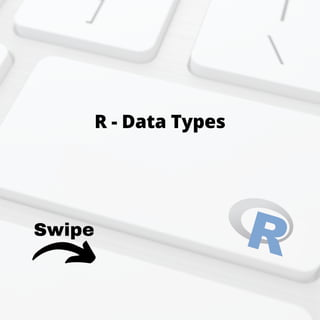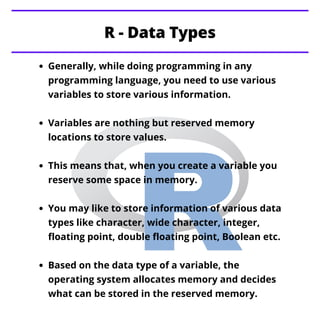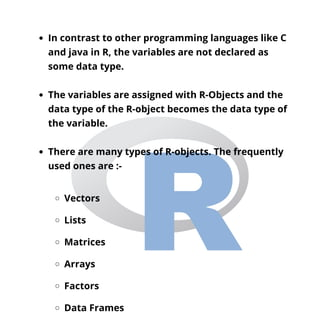Se está descargando tu SlideShare. ×
Anuncio
Anuncio
Anuncio
Anuncio
Anuncio
Anuncio
Anuncio
Anuncio
Anuncio
Anuncio
AnuncioAnuncio
Anuncio
Cargando en…3
×

1 de 13 Anuncio

# R data types

This presentation educates you about R - data types in detail with data type syntax, the data types are - Vectors, Lists, Matrices, Arrays, Factors, Data Frames.

For more topics stay tuned with Learnbay.

This presentation educates you about R - data types in detail with data type syntax, the data types are - Vectors, Lists, Matrices, Arrays, Factors, Data Frames.

For more topics stay tuned with Learnbay.

Anuncio
Anuncio

### R data types

1. 1. Swipe R - Data Types
2. 2. Generally, while doing programming in any programming language, you need to use various variables to store various information. Variables are nothing but reserved memory locations to store values. This means that, when you create a variable you reserve some space in memory. You may like to store information of various data types like character, wide character, integer, floating point, double floating point, Boolean etc. Based on the data type of a variable, the operating system allocates memory and decides what can be stored in the reserved memory. R - Data Types
3. 3. In contrast to other programming languages like C and java in R, the variables are not declared as some data type. The variables are assigned with R-Objects and the data type of the R-object becomes the data type of the variable. There are many types of R-objects. The frequently used ones are :- Vectors Lists Matrices Arrays Factors Data Frames
4. 4. When you want to create vector with more than one element, you should use c() function which means to combine the elements into a vector. Vectors When we execute the above code, it produces the following result # Create a vector. apple <- c('red','green',"yellow") print(apple) # Get the class of the vector. print(class(apple))  "red" "green" "yellow"  "character"
5. 5. A list is an R-object which can contain many different types of elements inside it like vectors, functions and even another list inside it. Lists When we execute the above code, it produces the following result # Create a list. list1 <- list(c(2,5,3),21.3,sin) # Print the list. print(list1) []  2 5 3 []  21.3 [] function (x) .Primitive("sin")
6. 6. A matrix is a two-dimensional rectangular data set. It can be created using a vector input to the matrix function. Matrices When we execute the above code, it produces the following result # Create a matrix. M = matrix( c('a','a','b','c','b','a'), nrow = 2, ncol = 3, byrow = TRUE) print(M) [,1] [,2] [,3] [1,] "a" "a" "b" [2,] "c" "b" "a"
7. 7. While matrices are confined to two dimensions, arrays can be of any number of dimensions. The array function takes a dim attribute which creates the required number of dimension. In the below example we create an array with two elements which are 3x3 matrices each. Arrays # Create an array. a <- array(c('green','yellow'),dim = c(3,3,2)) print(a)
8. 8. When we execute the above code, it produces the following result , , 1 [,1] [,2] [,3] [1,] "green" "yellow" "green" [2,] "yellow" "green" "yellow" [3,] "green" "yellow" "green" , , 2 [,1] [,2] [,3] [1,] "yellow" "green" "yellow" [2,] "green" "yellow" "green" [3,] "yellow" "green" "yellow"
9. 9. Factors are the r-objects which are created using a vector. It stores the vector along with the distinct values of the elements in the vector as labels. The labels are always character irrespective of whether it is numeric or character or Boolean etc. in the input vector. They are useful in statistical modeling. Factors are created using the factor() function. The n levels functions gives the count of levels. Factors
10. 10. # Create a vector. apple_colors <- c('green','green','yellow','red','red','red','green') # Create a factor object. factor_apple <- factor(apple_colors) # Print the factor. print(factor_apple) print(nlevels(factor_apple)) When we execute the above code, it produces the following result  green green yellow red red red green Levels: green red yellow  3
11. 11. Data frames are tabular data objects. Unlike a matrix in data frame each column can contain different modes of data. The first column can be numeric while the second column can be character and third column can be logical. It is a list of vectors of equal length. Data Frames are created using the data.frame() function. Data Frames # Create the data frame. BMI <- data.frame( gender = c("Male", "Male","Female"), height = c(152, 171.5, 165), weight = c(81,93, 78), Age = c(42,38,26) ) print(BMI)
12. 12. When we execute the above code, it produces the following result gender height weight Age 1 Male 152.0 81 42 2 Male 171.5 93 38 3 Female 165.0 78 26
13. 13. R - Decision making R - Loops R - Strings Stay Tuned with Topics for next Post